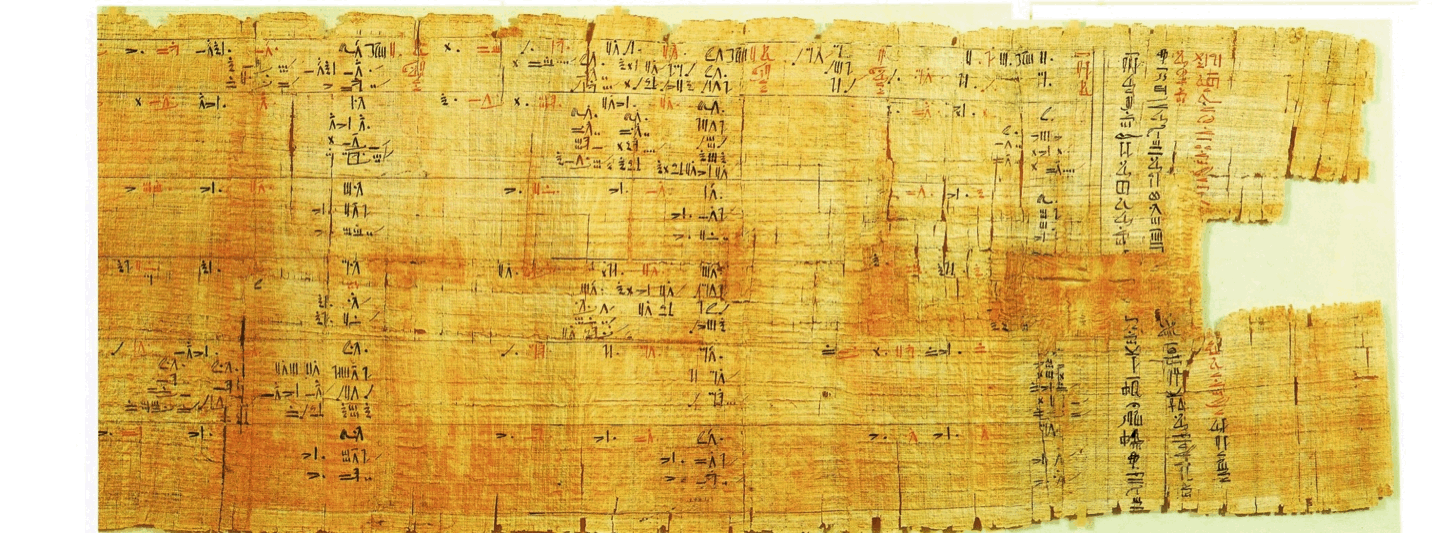# Zeta and Egyptian Fractions

What values of $\xi (-n)\quad$ (where n = odd number i.e, 1, 3, 5, 7) ..... can not be represented by Egyptian fractions or Egyptian fractions - x (where x is any integer) form. I am only considering Egyptian fraction with length greater than 1.

• For instance $\xi (-1)\quad$ = -1/12 = [(1/2)+(1/3)+(1/12)] - 1
• $\xi (-17)\quad$ = 1/2+1/3+1/9+1/625+1/816137+1/1046695702500 - 4
• $\xi (-11)\quad$ = 691/32760 = (1/48)+(1/3855)+(1/16838640)

However if you see $\xi (-3)\quad$ = 1/120 or $\xi (-7)\quad$ = 1/240 they can not be represented as Egyptian faction of length > 1 Also note that since we are only considering Egyptian fraction with length greater than 1 $\xi (-33)\quad$ which equals (1/12) -12635724796 also becomes part of this sequence

So If we start creating a sequence S(n) for which this is not possible then 3, 7 and 33 are first few candidates. I would like to learn more about this sequence. Also is there any way to know, for what values of n, $\xi (-n)\quad$ will be positive?Note by Vikram Pandya
5 years, 11 months ago

This discussion board is a place to discuss our Daily Challenges and the math and science related to those challenges. Explanations are more than just a solution — they should explain the steps and thinking strategies that you used to obtain the solution. Comments should further the discussion of math and science.

When posting on Brilliant:

• Use the emojis to react to an explanation, whether you're congratulating a job well done , or just really confused .
• Ask specific questions about the challenge or the steps in somebody's explanation. Well-posed questions can add a lot to the discussion, but posting "I don't understand!" doesn't help anyone.
• Try to contribute something new to the discussion, whether it is an extension, generalization or other idea related to the challenge.

MarkdownAppears as
*italics* or _italics_ italics
**bold** or __bold__ bold
- bulleted- list
• bulleted
• list
1. numbered2. list
1. numbered
2. list
Note: you must add a full line of space before and after lists for them to show up correctly
paragraph 1paragraph 2

paragraph 1

paragraph 2

[example link](https://brilliant.org)example link
> This is a quote
This is a quote
    # I indented these lines
# 4 spaces, and now they show
# up as a code block.

print "hello world"
# I indented these lines
# 4 spaces, and now they show
# up as a code block.

print "hello world"
MathAppears as
Remember to wrap math in $$ ... $$ or $ ... $ to ensure proper formatting.
2 \times 3 $2 \times 3$
2^{34} $2^{34}$
a_{i-1} $a_{i-1}$
\frac{2}{3} $\frac{2}{3}$
\sqrt{2} $\sqrt{2}$
\sum_{i=1}^3 $\sum_{i=1}^3$
\sin \theta $\sin \theta$
\boxed{123} $\boxed{123}$